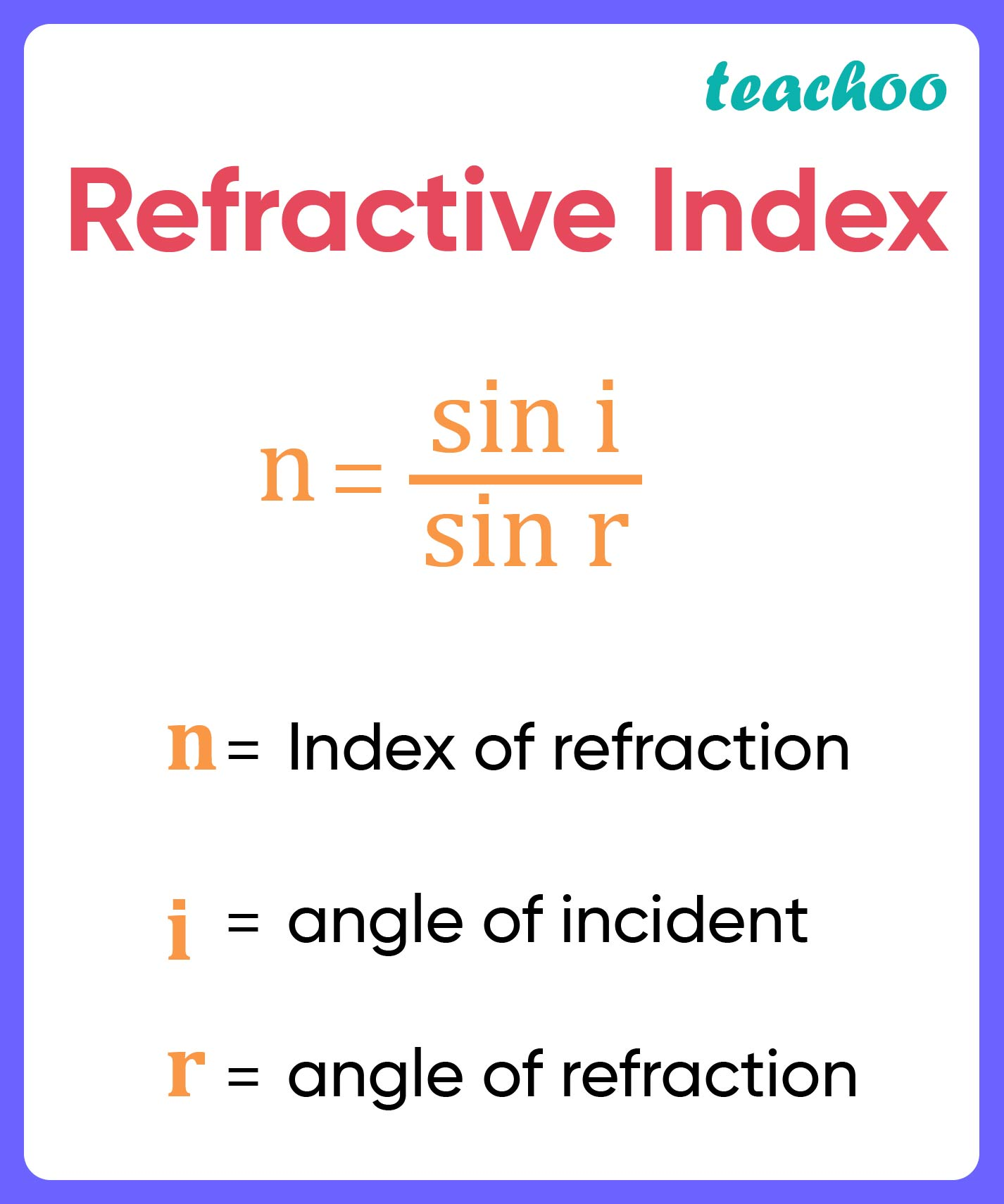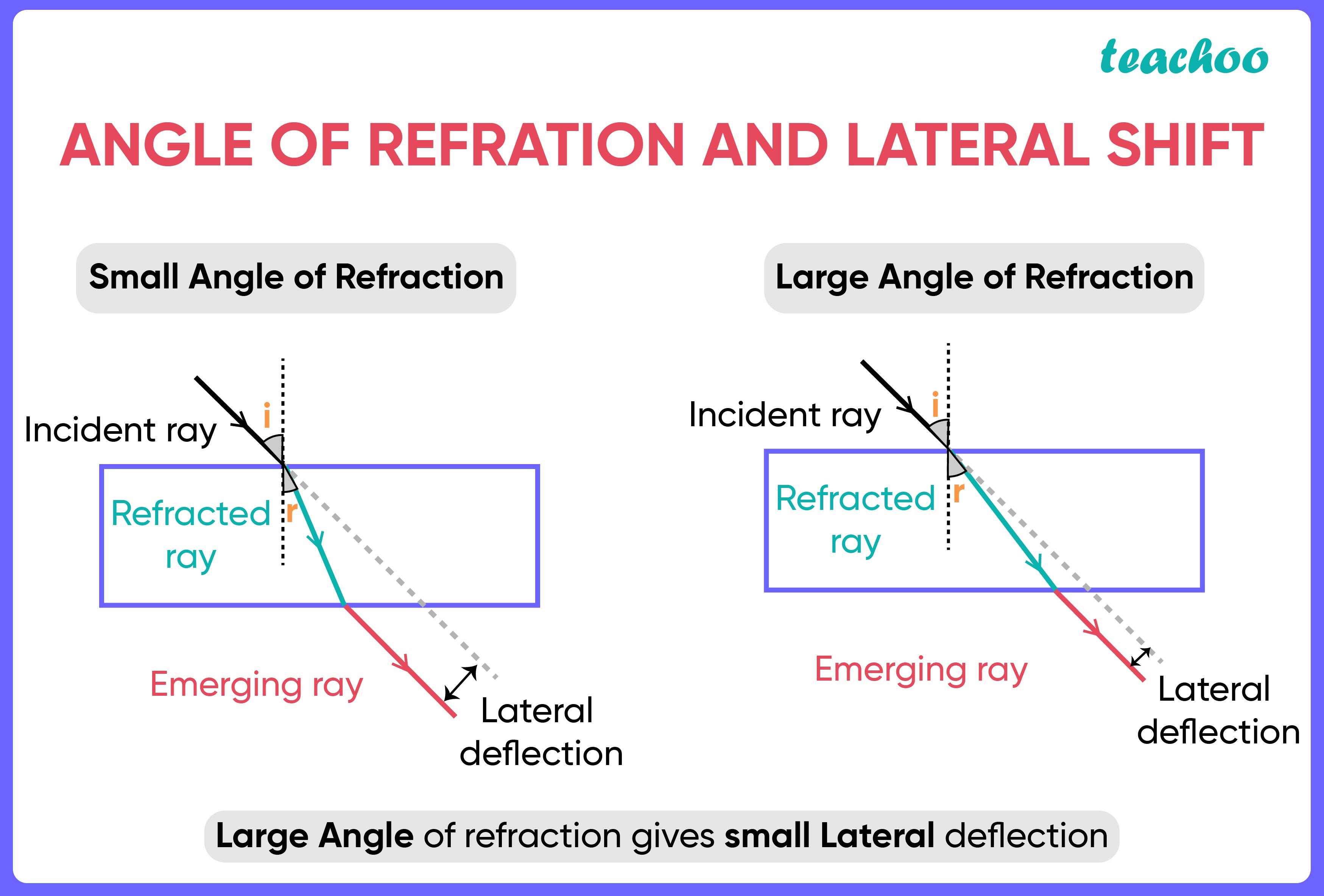Solutions - CBSE Class 10 Sample Paper for 2022 Boards - Science [MCQ]

Class 10
Solutions to CBSE Sample Paper - Science Class 10

## (D) there is not enough information to comment on any of the above statements

Now,

• Greater the refractive index , smaller the angle of refraction• If we decrease the refractive index, the angle of refraction increases
• This lateral shift of rays will be less, shift will be less because of angle of refraction is becoming equal to angle of incidenceSo, the correct answer is (a)

Learn in your speed, with individual attention - Teachoo Maths 1-on-1 Class